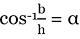# valarray acos() Function in C++ with Examples

C++ valarray acos() Function: Here, we will learn about the acos() function, its usages, syntax and examples.
Submitted by Shivang Yadav, on May 20, 2022

The valarray class in C++ is a special container that is used for holding elements like an array and performing operations on them.

Mathematical Arc Cosine

Mathematically, arc cosine is the inverse of cosine function. The arc cosine often known as cos inverse is used to evaluate the value of angle using the given ratio of side adjacent to the angle and hypotenuse.

Arc Cosine is denoted as cos-1 and formulated as,Where,

• b is the length of side adjacent to the angle
• h is the length of hypotenuse
• α is the angle

## std::acos(std::valarray) Function

The acos() function in the valarray class is a function used for creating a valarray consisting of elements calculated as cos-1 for each element of the original valarray.

Syntax:

```template< class T >
valarray<T> acos( const valarray<T>& va );
```

Parameter(s): It takes one parameter the valarray on which the mathematical operation is to be applied.

Return Value: It returns a valarray consisting of cos-1 value of each element of the initial valarray.

This is all the basics about the valarray function. Moving ahead, we are now creating programs to test value on the acos() function and results on different sets of values.

## C++ valarray acos() Function Example 1:

```#include <iostream>
#include <valarray>
using namespace std;

int main()
{
// Declaring valarray
valarray<int> myvalarr = { 16, 314, 0, 1, 2 };

// Printing the elements of valarray
cout << "The elements of orignal valarray are : ";
for (int& ele : myvalarr)
cout << ele << " ";

valarray<int> acosvalarray = acos(myvalarr);
cout << "\nThe elements of acos valarray are : ";
for (int& ele : acosvalarray)
cout << ele << " ";

return 0;
}
```

Output:

```The elements of orignal valarray are : 16 314 0 1 2
The elements of acos valarray are : -2147483648 -2147483648 1 0 -2147483648
```

Note: The value -2147483648 denotes that the value does not exist. As mathematically the value passed in cos-1 should lie between -1 and 1.

## C++ valarray acos() Function Example 2:

```#include <iostream>
#include <valarray>
using namespace std;

int main()
{
// Declaring valarray
valarray<double> myvalarr = { 1.6, -0.5, 0, 1, -1 };

// Printing the elements of valarray
cout << "The elements of orignal valarray are : ";
for (double& ele : myvalarr)
cout << ele << " ";

valarray<double> acosvalarray = acos(myvalarr);
cout << "\nThe elements of acos valarray are : ";
for (double& ele : acosvalarray)
cout << ele << " ";

return 0;
}
```

Output:

```The elements of orignal valarray are : 1.6 -0.5 0 1 -1
The elements of acos valarray are : nan 2.0944 1.5708 0 3.14159
```

Preparation

What's New

Top Interview Coding Problems/Challenges!